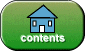created: 07/27/2018

# Quiz on More JavaFX

Instructions: For each question, choose the single best answer. Make your choice by clicking on its button. To grade the quiz, click on the button at the bottom.

1. To be part of the scene graph an object must inherit from what class?

A.    Node

B.    Shape

C.    Parent

D.    Pane

2. What is the parent class of `Circle`, `Line`, `Rectangle` (and others)?

A.    Scene

B.    Shape

C.    Object

D.    Control

3. What are the four parameters of one of the `Ellipse` constructors?

A.    upperLeftX, upperLeftY, lowerRightX, lowerRightY

D.    centerX, centerY, fillColor, strokeColor

4. An `Arc` is a part on an `Ellipse`. How is the part described?

A.    start angle in degrees, end angle in degrees

C.    start angle in degrees, length of the arc in degrees counter-clockwise

D.    start angle in degrees, length of the arc in degrees clockwise

5. What class represents a sequence of connected line segments?

A.    Polyline

B.    Polygon

C.    Polyphemus

D.    Multiline

6. What `Shape` class is used to put words in the window?

A.    String

B.    Character

C.    Word

D.    Text

7. How are the number of sides specified in the `Polygon` constructor?

A.    The number of sides is the number of X-Y pairs of vertex coordinates.

B.    The first parameter of the constructor specifies the number of sides.

C.    The number of sides is the length of the array parameter

D.    The number of sides is the number of parameters.

8. The `setRotate(double value)` method works with any `Node` or descendant class. What does its parameter `value` specify?

A.    The number of degrees of clockwise rotation of the `Node` about its center.

B.    The number of degrees of counter-clockwise rotation of the `Node` about its center.

C.    The number of radians of clockwise rotation of the `Node` about its center.

D.    The number of radians of counter-clockwise rotation of the `Node` about its center.

9. If a transformation is applied to a `Group` what happens to the `Nodes` that are contained in it?

A.    They are unaffected.

B.    The transformation is applied to all nodes in the group.

C.    The transformation is applied only to the `Shape`s in the group.

D.    The transformation is applied to all nodes in the group except for those that are in groups contained in this group.

10. What type of `Pane` automatically centers a `Node` put into it?

A.    `HBox`

B.    `VBox`

C.    `FlowPane`

D.    `StackPane`

The number you got right:       Percent Correct:       Letter Grade:If you have returned here from another page, or have re-loaded this page, you will need to click again on each of your choices for the grading program to work correctly. You may want to press the SHIFT KEY while clicking to clear the old answers.